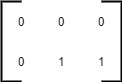New update is available. Click here to update.

# Leftmost Column With At Least A One

Last Updated: 24 Feb, 2021
Difficulty: Moderate

## PROBLEM STATEMENT

#### However, you can not access the binary matrix directly. The function dimensions() return an array of two elements [m, n] which denote the number of rows and the number of columns in the matrix respectively. The function get(x, y) returns the element of the matrix at the index (x, y).

##### Note :
``````We are following 0-based indexing for this problem. The get method should be called for a max of 2000 times, and the dimensions method should be called only once. Submissions making more than 2000 calls to the get method and more than one call to the dimensions will be judged as the wrong answers.
``````
##### For Example :
``````If the BinaryMatrix has 2 rows and 3 columns and the matrix is {{0, 0, 0}, {0, 1, 1}}. Then, both the second and the third columns contain a 1, but the second column is the leftmost column. Hence, the answer is 1 (0-based indexing).
````````````Note that, here, the BinaryMatrix is given as an example. In the actual problem, you have to access the elements of the matrix by using the BinaryMatrix.get() method.
``````
##### Input format :
``````The first line contains an integer 'T', which denotes the number of test cases or queries to be run. Then, the T test cases follow.

The first line of each test case contains two space-separated integers M and N, denoting the number of rows and the number of columns in the matrix, respectively.

Then M lines follow. Each line contains N space-separated integers denoting elements of the matrix.

Note that the input is given for reference. You can’t access the elements of the matrix directly. You have to use the get method to do so.
``````
##### Output format :
``````For each test case, print the leftmost index (0-based indexing) of the column such that it contains at least one 1. If such a column doesn’t exist in the matrix, then return -1.

Output for each test case will be printed in a separate line.

If the program calls the get method more than 2000 times or the dimensions function more than once, then it will be judged as a wrong answer, and the program stops instantly.
``````
##### Note :
``````You do not need to print anything. It has already been taken care of. Just implement the given function.
``````
##### Constraints :
``````1 <= T <= 5
1 <= M <= 1000
1 <= N <= 1000
0 <= BinaryMatrix[i][j] <= 1

Time limit: 1 second
``````
SIMILAR PROBLEMS

Search In A Sorted 2D Matrix

Posted: 23 Nov, 2022
Difficulty: Moderate

Spiral Matrix

Posted: 24 Nov, 2022
Difficulty: Easy

Three Sum

Posted: 24 Nov, 2022
Difficulty: Moderate

Sort 0s, 1s, 2s

Posted: 24 Dec, 2022
Difficulty: Easy

Fake Coin Problem

Posted: 24 Dec, 2022
Difficulty: Easy